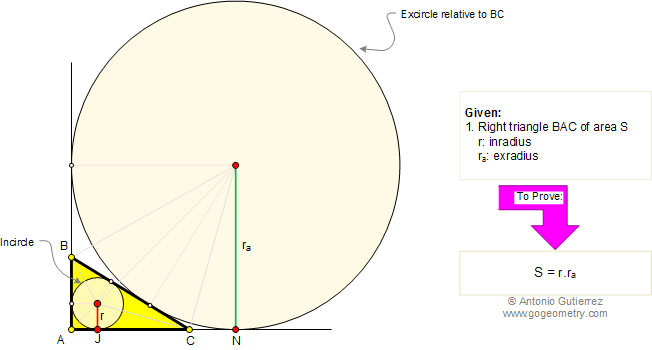Problem 206. Area of a Right Triangle Area, Inradius, Exradius relative to the hypotenuse
 In the figure below, BAC is a right triangle of area S. If r is the inradius and ra is the exradius relative to the hypotenuse BC, prove that: S = r.ra. View or post a solution.Home | Geometry | Search | Problems | 201-210 | Area of a Triangle | Right Triangle Area | View or post a solution | Email